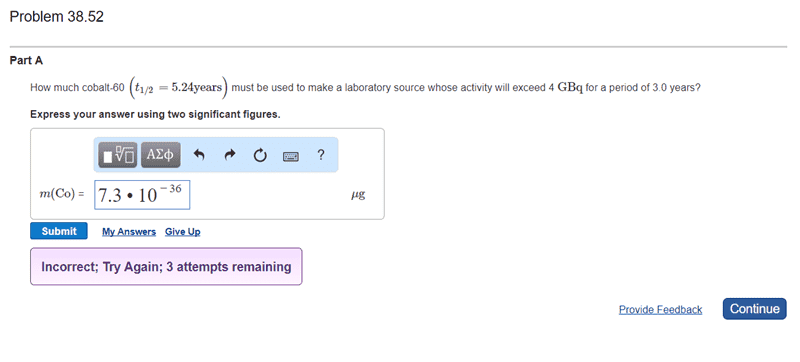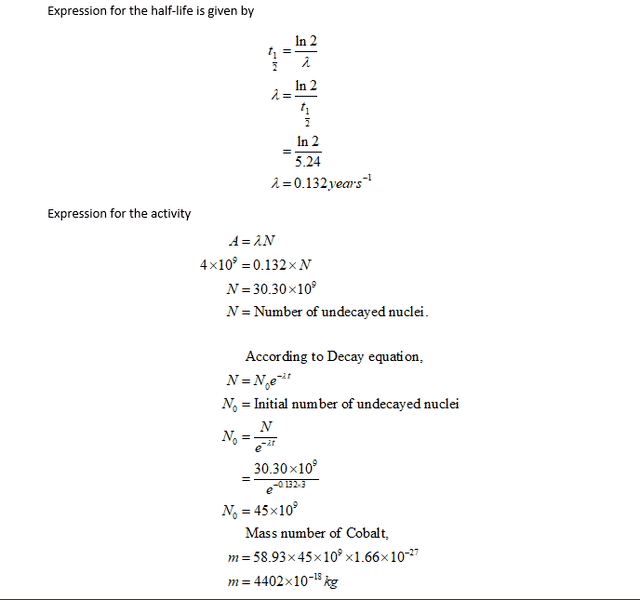# Find Mass given half life

• Noreturn
In summary, the conversation is about converting a given value into micrograms and finding the initial number of nuclei using the activity equation A(t) = A0 e-λt. The conversation also touches on the units of bequerel and how to properly convert between different units.

## Homework Statementhttp://d2vlcm61l7u1fs.cloudfront.net/media/f62/f62b6919-e60f-405b-81fb-2323ceeb03ee/phpsL4bVl.png

## Homework EquationsSo I need that in micrograms tho. So 4402*10^-18/1000=4.4*10^-18kg. or 4.4*10^-12 micrograms

that stills say it's wrong tho.

#### Attachments

Perhaps you should use the activity equation A(t) = A0 e-λt to find the initial activity A0 and convert that to the initial number of nuclei.

Didn't we do that at the bottom of the image?

Watch units. A bequerel is defined how?

Oh! So:
4402 * (10^(-18)) kg = 4.40200 × 10-6 micrograms

BUT bequerel is s^-1 @ 10^-6 so answer is 4.4micrograms?

Noreturn said:
Didn't we do that at the bottom of the image?
Not really. To reinforce what @TSny posted, assuming that you found A0, what is the number for λ in N0 = λ A0 when A0 has Bq units?

Noreturn said:
BUT bequerel is s^-1 @ 10^-6 so answer is 4.4micrograms?

I was not able to figure this out. Any help on where I went wrong is appreciated. So I'm guessing I did the -.1322 wrong based on what you guys have mentioned.

Look at the equation A = λ N. Put in the numbers including the units attached to each number. Then you will see what is going on.

Do I need to divide by Avogadro constant?

Noreturn said:
Do I need to divide by Avogadro constant?
Not in this equation. Just do what I suggested.

4*10^9 Bq =.132yr^-1*N

or

4*10^9Bq = 4.18291693 × 10-9 Bq * N

N= 9.56*10^17

9.56*19^17/e^(.132*3) = 6.43*10^17

(6.43*10^17)(1.66*10^-27)(58.93) =6.28*10^-8kg or 6.3ug

Last edited:
That's better but still incorrect. What does N= 9.56*10^17 represent? What about 6.43*10^17? What number is that? If N is the number of undecayed nuclei, after 3 years (when the activity is 4*10^9Bq) and 6.43*10^17 is the initial number of undecayed nuclei, which one should be the larger number? Also, what are the units of 1.66*10^-27 and 58.93?

So Muliplying 1.66*10^-27 and 58.93 converts mass number of Cobalt from amu to kg.

The Initial should be bigger.

Just realized I may have had it right but I forgot to convert the kg to g. So my answer should have been 6.28ug

Noreturn said:
The Initial should be bigger.
I agree, but when you start with the equation A = λ N, then you say A = 4*10^9Bq, that "A" is the final A and the number N = 9.56*10^17 that you get from it is the final N. So, what is the initial N that should be larger than 9.56*10^17? That's why I suggested that you find the initial activity in post #2.
Noreturn said:
Just realized I may have had it right but I forgot to convert the kg to g. So my answer should have been 6.28ug
I prefer to look at it this way: If you have N atoms of atomic weight AW, the mass of the sample is given by
$$m=AW \left(\frac{grams}{mole} \right)\times N (atoms) \times \frac{1}{N_{Avog.}} \left(\frac{mole}{atoms} \right)=AW\times \frac{N}{N_{Avog.}}(grams)$$
It's not a coincidence that the inverse of Avogadro's number (1.66×10-24) matches the mass of a proton (or neutron) in grams, 1.67×10-24.